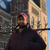# New to Qlik Sense

If you’re new to Qlik Sense, start with this Discussion Board and get up-to-speed quickly.

Announcements
cancel
Showing results for
Did you mean:Contributor

## Autocalendar.MonthsAgo

Hello,

I am very new to Qlik, using QlikSense desktop. I have two year (2016, 2017) daily sales data like below

Date / Sales

01.10.16 / 100

05.10.16 / 50

04.03.17 / 20

05.08.17 / 60

...

Today () = 6/11/17

I am just trying to find Total Sales for the Selected Month and Previous Month. Below is my script

My Dimension is Date.autoCalendar.YearMonth

Count({1<[Date.autoCalendar.MonthsAgo]={\$(vMonthsAgo)}, [Date.autoCalendar.YearsAgo]={\$(vYearsAgo)}>}[Sales])

vMonthAgo=(Year(Today())-Year(Max([Stay Date])))*12 + Month(Today())- Month(Max([Stay Date]))+1

vYearsAgo =Year(Today())- Year(AddMonths(Max([Stay Date]),-1))

It works well when I select a months in 2017 but it gives 0 when I select a month in 2018?

Can someone help me with this?

Thank you

1 Solution

Accepted SolutionsPartner - Champion II

It's a matter of choice; when you work with qlik ; you'll love it; and when you'll do, you'll want to build your own expressions and have full control over everything.

This does not ommit the fact that the auto calendar is a great feature.

please refer to this video : (other ones can be found)

Creating calendar measures - Qlik Sense - YouTube

mto‌, if you any other sources that can help him, please do shareHope this helps !

Omar BEN SALEM.

4 RepliesChampion

omarbensalem‌b I'll let you answer himPartner - Champion II

Sure.

Since you're new to Qlik Tolga, I'll try to explain to you the time functions step by step:

Let's suppose you have a date field (you don't have to create variables); only a date field will do the job.

And let's suppose you've created a calendar in your script so you have Year,Month, Quarter and Week fields

YTD: if we select 12/04/2016: YTD will return our measure from 01/01/2016 to 12/04/2016

How we do that?

Suppose our measure is : sum(Sales)

1)First changes: sum({<date=,Year=,Month=,Quarter=>}Sales)

We add these to force Qlik to not take into consideration our selection of date for example.

Let me explain in better words, if you don't write the "date=" and select the date 12/04/2016; Qlik will filter all the data to that selected point and then return the sum(Sales) for the day 12/04/2016.

To prohibit this, we must write the date=.

2) Second change: sum({<date=,Year=,Month=,Quarter=, date={">=\$(=YearStart(Max(date)))<=\$(=Max(date))"}>}Sales)

Let explain this : date={">=\$(=YearStart(Max(date)))<=\$(=Max(date))"}

We want to work from date=01/01/2016 to the selected date=12/04/2016 right?

So we're working with the field :

a) date={    }

b) Now we wanna this date to be <=selected date which is max(date) ;

max(date) is a function so it needs an "=" sign:

=max(date)

when we have a '=' we add the \$ (before each calculation) : \$(=max(date) ) => this is 12/04/2016

Now we add the <= so we'll have :  <=\$(=max(date) )

for the second part, we want our date to be >=01/01/2016 which is the start of the year:

a) same approach, we use the YearStart function that returns the start of the selected year: >=\$(=YearStart(Max(date)))

Now our expression is : from : date={    }

to : date={>=\$(=YearStart(Max(date)))<=\$(=Max(date))}

Now, in a set expression, if we wanna work with the year 2016 for example which is numeric: we call it without quotes:

Year={2016}

If we wanna focus on a country, Tunisia for example which is a string: we call it with quotes

Country={'Tunisia'}

In our case, we focusing on a range of dates created by an expression, so we surround it by double quotes:

date={">=\$(=YearStart(Max(date)))<=\$(=Max(date))"}

Final expression for YTD:

sum({<date=,Year=,Month=,Quarter=, date={">=\$(=YearStart(Max(date)))<=\$(=Max(date))"}>}Sales)

Same approach for MTD:

sum({<date=,Year=,Month=,Quarter=, date={">=\$(=MonthStart(Max(date)))<=\$(=Max(date))"}>}Sales)

So, if we wanna focus on the YTD-1, we wanna alter this part:

DATE={">=\$(=YearStart(Max(DATE)))<=\$(=Max(DATE))"}

to do so, we want to situate our self in the previous year, to do that, their is a function called addYears.

How we use it? addYears('04/12/2017',-1) = 04/12/2016;

So our expression will become:

With this, if the max(Date) in 2017 is 24/03/2017 (like in your case)

The YTD-1 will return the NET_AMOUNT from 01/01/2016 to 24/03/2016 .

Hope this helps,

Omar,Contributor
Author

Thanks a lot omarbensalem, surely it will help; since you have explained it step by step. I've tried and it worksBut how about this "autocalendar" property? I have assumed it would make life far easier for anyone who is working with dates; but I couldn't see much resources about it in the forum. Any specific reason that you are not using it?

thank youPartner - Champion II

It's a matter of choice; when you work with qlik ; you'll love it; and when you'll do, you'll want to build your own expressions and have full control over everything.

This does not ommit the fact that the auto calendar is a great feature.

please refer to this video : (other ones can be found)

Creating calendar measures - Qlik Sense - YouTube

mto‌, if you any other sources that can help him, please do shareHope this helps !

Omar BEN SALEM.